stat_slice {ggbio} R Documentation

## Slice Rle/RleList to view them as bar or heatmap.

### Description

Slice Rle/RleList to different view by set lower or other parameters, then view summary for all those viewed region.

### Usage

```## S4 method for signature 'Rle'
stat_slice(data, ...,
xlab, ylab, main,
na.rm = FALSE,
geom = NULL,
lower=-Inf, upper=Inf,
includeLower=TRUE, includeUpper=TRUE,
rangesOnly = FALSE,
type = c("viewSums","viewMins",
"viewMaxs", "viewMeans"))

## S4 method for signature 'RleList'
stat_slice(data, ...,
xlab, ylab, main,
indName = "sample",
na.rm = FALSE,
geom = NULL,
lower=-Inf, upper=Inf,
includeLower=TRUE, includeUpper=TRUE,
rangesOnly = FALSE,
type = c("viewSums","viewMins",
"viewMaxs", "viewMeans"))
```

### Arguments

 `data` a `data.frame` or `Rle` or `RleList` object. `...` arguments passed to aesthetics mapping. `xlab` x label. `ylab` y label. `main` title. `indName` when faceted by a `RleList`, name used for labeling faceted factor. Default is 'sample'. `geom` geometric types. `type` statistical summary method used within bins, shown as bar height or heatmap colors. `na.rm` logical value, default `FALSE`, passed to function like `viewMaxs` for statistical summary computation. `lower` passed to `slice`. `upper` passed to `slice`. `includeLower` passed to `slice`. `includeUpper` passed to `slice`. `rangesOnly` passed to `slice`.

a ggplot object.

Tengfei Yin

`slice`

### Examples

``````library(IRanges)
lambda <- c(rep(0.001, 4500), seq(0.001, 10, length = 500),
seq(10, 0.001, length = 500))
xVector <- rpois(1e4, lambda)
xRle <- Rle(xVector)
xRleList <- RleList(xRle, 2L * xRle)
ggplot(xRle) + stat_slice(lower = 5)
``````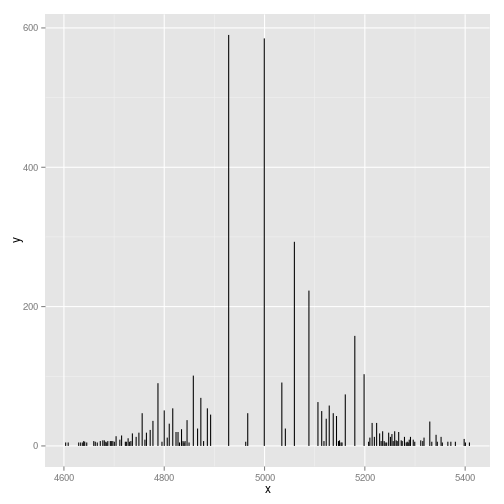``````ggplot(xRle) + stat_slice(lower = 5, geom = "bar")
``````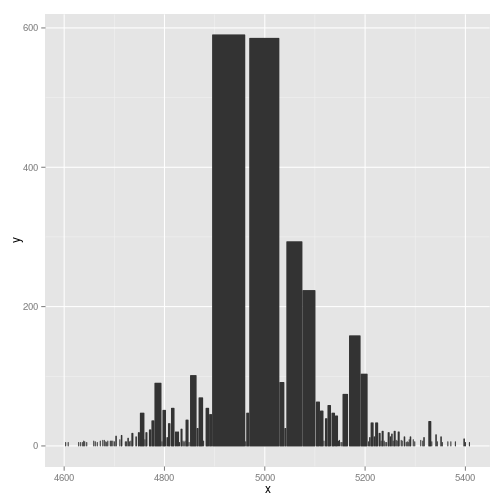``````ggplot(xRle) + stat_slice(lower = 5, geom = "heatmap")
``````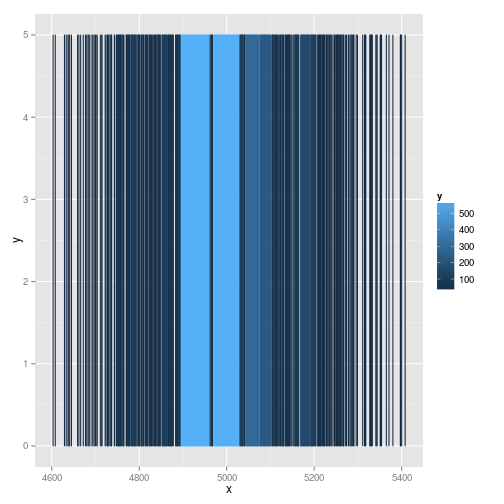``````p1 <- ggplot(xRle) + stat_slice(type = "viewMeans", lower = 5,
geom = "bar")
p2 <- ggplot(xRle) + stat_slice(type = "viewSums", lower = 5,
geom = "bar")
## y scale are different.
tracks(viewMeans = p1, viewSums = p2)
``````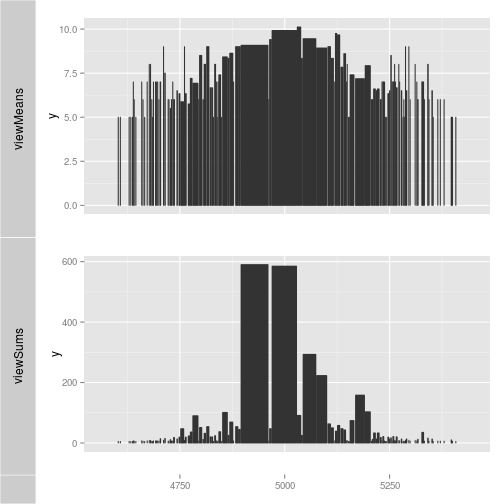``````ggplot(xRleList) + stat_slice(lower = 5)
``````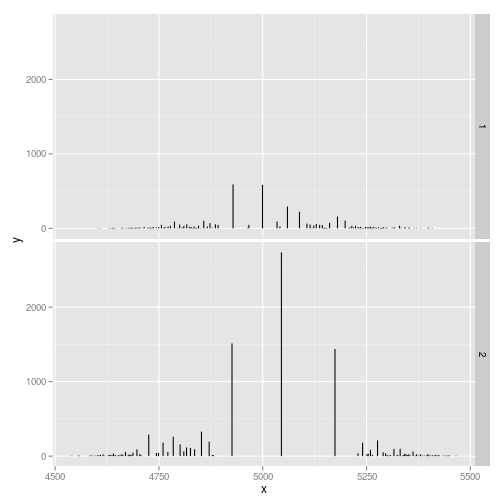``````ggplot(xRleList) + stat_slice(lower = 5, geom = "bar")
``````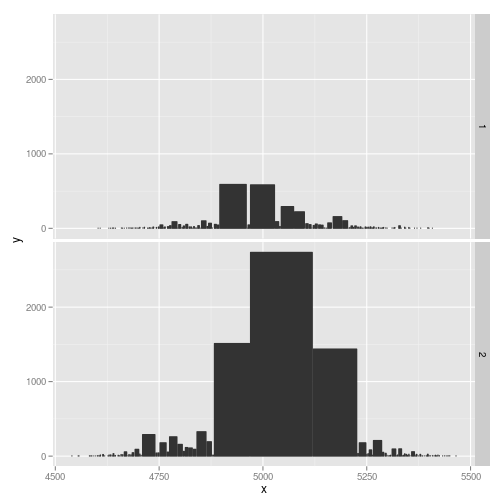``````ggplot(xRleList) + stat_slice(lower = 5, geom = "heatmap")
``````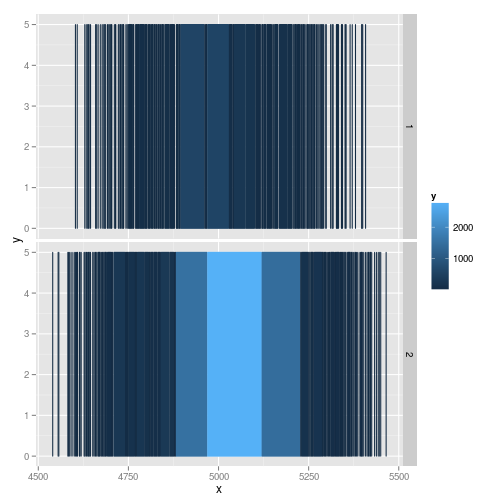``````p1 <- ggplot(xRleList) + stat_slice(type = "viewMeans", lower = 5,
geom = "bar")
p2 <- ggplot(xRleList) + stat_slice(type = "viewSums", lower = 5,
geom = "bar")
## y scale are different.
tracks(viewMeans = p1, viewSums = p2)
``````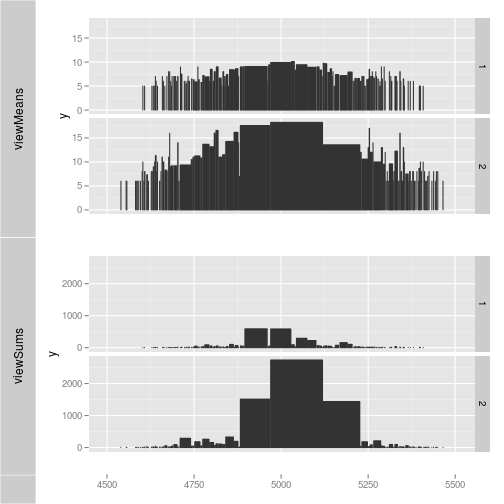[Package ggbio version 1.5.20 ]